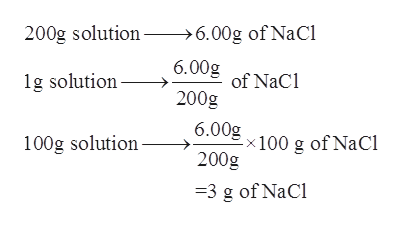# The % m/m of a solution made by dissolving 6.00 g NaCl to make 200. g of solution is _______% m/m.

Question
40 views

The % m/m of a solution made by dissolving 6.00 g NaCl to make 200. g of solution is _______% m/m.

check_circle

Step 1

The term molarity is used to express the number of moles of solution which are dissolved in one liter of the given solution.

Step 2

The mass percent of a given solution which is denoted by (% m/m) can be calculated by estimating the grams of solute which are present in 100 g of the given solution. The mathematical formula is as follows:

Step 3

It is given that 200 g of solution contains 6.00 g o...help_outlineImage Transcriptionclose200g solution >6.00g of NaCl 6.00g 1g solution of NaCl 200g 6.00g x 100 g of NaCl 200g 100g solution =3 g of NaCl fullscreen

### Want to see the full answer?

See Solution

#### Want to see this answer and more?

Solutions are written by subject experts who are available 24/7. Questions are typically answered within 1 hour.*

See Solution
*Response times may vary by subject and question.
Tagged in

### Physical Chemistry